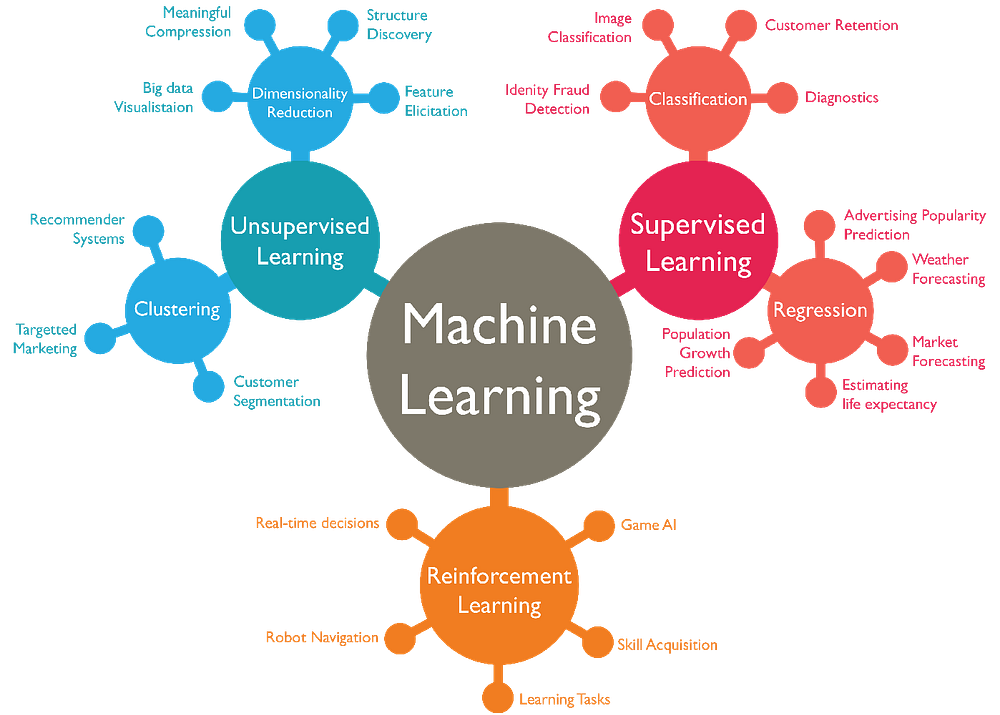# Machine learning for grandmothers

Source: Deep Learning on Medium## Supervised Algorithms

A supervised algorithm is is one in which the input data is required to be labeled with the expected outputs of these, so that through mathematical operations you can approximate and adjust the parameters or weights within the algorithm and finally obtain the expected outputs having only the input data.

However, these types of algorithms can also be divided into two types depending on the type of tags that the data has, such as classification and regression.

The classification algorithms are those that are designed to fit labels in categories, such as an algorithm to identify the color of an object between: white, green, blue, etc. having only the image pixel data.

On the other hand, regression algorithms are those that are designed to adjust data that have continuous or discrete numerical values as labels, in which the outputs depend on one or several variables such as: an algorithm to predict the stock’s price.

## Unsupervised Algorithms

An unsupervised algorithm is one in which the input data is not labeled, so it is up to the algorithm to adjust the commonalities that it considers to classify the input data into some category. An example of this type of algorithm is market segmentation to classify the types of customers a business has.

An advantage of this type of algorithm is that, of course, the data is not usually labeled, therefore, it is usually ideal for a first analysis, since the labeling process can be expensive in some situations.

## Reinforcement learning

They are a type of algorithms where you learn through a behavioral efoque, in which the algorithm tries to accumulate the greatest amount of reward.